Next: Velocity averaging lemmas () Up: Kinetic Formulations and Regularity Previous: Kinetic Formulations and Regularity

# Regularizing effect in one-space dimension

We consider the convex conservation law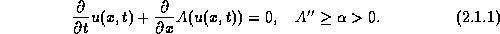Starting with two values at the different positions,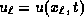and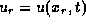, we trace these values by backward characteristics. They impinge on the initial line at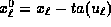and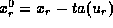, respectively. Since the characteristics of entropy solutions of convex conservation laws cannot intersect, one finds that the ratio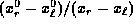remains positive for all time. After rearrangement this yields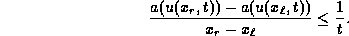Thus we conclude that the velocity of a(u) satisfies the Oleinik's one-sided Lip condition,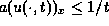. Thanks to the convexity of A, we obtain the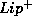bound on u itself,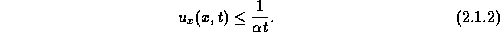We recall thatbound (2.1.2) served as the cornerstone for the Lip' convergence theory outlined in Lecture IV. Here we focus on the issue of it regularity. Granted (2.1.2), it follows that the solution operator associated with convex conservation laws,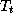, has a nonlinear regularizing effect, mapping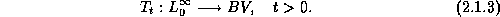Indeed, for uniformly bounded initial data,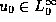, with compact support of size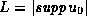, one obtains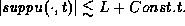Thebound (2.1.2) then yields an upper bound on the positive variation,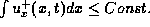; since the sum of the positive and negative variations is bounded,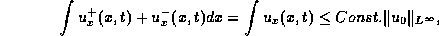it follows that their difference is also bounded,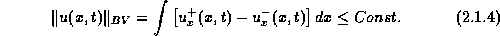Observe that no regularity is 'gained' in the linear case, where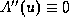. Indeed, the compactness asserted in (2.1.3) is a purely nonlinear regularizing phenomenon which reflects the irreversibility of nonlinear conservation laws, due to loss of entropy (information) across shock discontinuities. Here, nonlinearity is quantified in terms of convexity; in the prototype example of the inviscid Burgers' equation,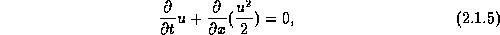one finds a time decay,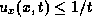. Tartar  proved this regularizing effect for general nonlinear fluxes -- nonlinear in the sense of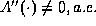.

The situation with multidimensional equations, however, is less clear. Consider the 'two-dimensional Burgers' equation', analogous to (2.1.5)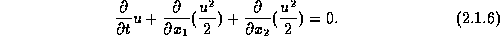Since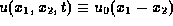is a steady solution of (2.1.6) for any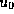, it follows that initial oscillations persist (along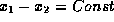), and hence there is no regularizing effect which guarantee the compactness of the solution operator in this case. More on oscillations and discontinuities can be found in Tartar's review .Next: Velocity averaging lemmas () Up: Kinetic Formulations and Regularity Previous: Kinetic Formulations and Regularity